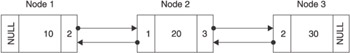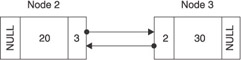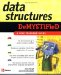# Linked List Queue Using C

## Linked List Queue Using C++

Now that you understand how to create a queue using a linked list, let s assemble all the pieces and build a working queue in C++. Programmers organize an application into several files, each containing a distinct component of the application.

The application is called QueueLinkedListDemo , and it uses a linked list to create a queue, as shown in the next code. The application begins by declaring an instance of the QueueLinkedList class using the new operator. It then declares a pointer to an instance of the QueueLinkedList . The pointer is called queue , which is assigned a reference to the instance created by the new operator.

The enqueue() member function is then called three times, each time another node is placed on the queue. The queue shown in Figure 8-4 depicts the queue after the last time the enqueue() method is called.Figure 8-4: The queue after all three values are placed on the queue.

The dequeue() member function is then called to remove the first node from the queue and display its data member on the screen. Figure 8-5 shows the queue after the dequeue() member function is called.Figure 8-5: The queue after the dequeue() member function is called

The last statement in the program removes the queue from memory.

Each of the remaining components of the application was discussed in the previous section.

` //QueueLinkedListDemo.cpp #include <iostream> using namespace std; void main(){  QueueLinkedList* queue = new QueueLinkedList();  queue->enqueue(10);  queue->enqueue(20);  queue->enqueue(30);  cout << queue->dequeue() << endl;  delete queue; } //LinkedList.h typedef struct Node {  struct Node(int data)  {  this->data = data;  previous = NULL;  next = NULL;  }  int data;  struct Node* previous;  struct Node* next; } NODE; class LinkedList {  protected:  NODE* front;  NODE* back;  public:  LinkedList();  ~LinkedList();  void appendNode(int);  void displayNodes();  void displayNodesReverse();  void destroyList(); }; //LinkedList.cpp #include "LinkedList.h" LinkedList::LinkedList() {  front = NULL;  back = NULL; } LinkedList::~LinkedList() {  destroyList(); } void LinkedList::appendNode(int data) {  NODE* n = new NODE(data);  if(front == NULL)  {  back = n;  front = n;  }  else  {  back->next = n;  n->previous = back;  back = n;  } } void LinkedList::displayNodes() {  cout << "Nodes:";  NODE* temp = front;  while(temp != NULL)  {  cout << " " << temp->data;  temp = temp->next;  } } void LinkedList::displayNodesReverse() {  cout << "Nodes in reverse order:";  NODE* temp = back;  while(temp != NULL)  {  cout << " " << temp->data;  temp = temp->previous;  } } void LinkedList::destroyList() {  NODE* temp = back;  while(temp != NULL)  {  NODE* temp2 = temp;  temp = temp->previous;  delete temp2;  }  back = NULL;  front = NULL; } //QueueLinkedList.h #include "LinkedList.h" class QueueLinkedList : public LinkedList {  public:  QueueLinkedList();  virtual ~QueueLinkedList();  void enqueue(int);  int dequeue();  bool isEmpty(); }; //QueueLinkedList.cpp #include "QueueLinkedList.h" QueueLinkedList::CQueueLinkedList() { } QueueLinkedList::~CQueueLinkedList() { } void QueueLinkedList::enqueue(int x) {  appendNode(x); } int QueueLinkedList::dequeue() {  if(isEmpty())  {  return -1;  }  int retVal = front->data;  NODE* temp = front;  if(front->next == NULL)  {  back = NULL;  front = NULL;  }  else  {  front = front->next;  front->previous = NULL;  }  delete temp;  return retVal; } bool QueueLinkedList::isEmpty() {  if(front == NULL)  {  return true;  }  else  {  return false;  } } `Data Structures Demystified (Demystified)
ISBN: 0072253592
EAN: 2147483647
Year: 2006
Pages: 90

Similar book on Amazon